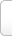﻿ 没组词_没字可以怎么组词_能组什么词_有哪些

# 没 字可以怎么组词

• 没略
• 没地
• 没滑
• 没脊骨
• 没掂三
• 没成捆
• 没阶
• 没规矩
• 没过
• 没乱杀
• 没情没绪
• 没女
• 没皮没脸
• 没口
• 没张致
• 没溺
• 没祥
• 没分寸
• 没里没外
• 没兴
• 没眉没眼
• 没佯
• 没完没了
• 没心程
• 没交涉
• 没日没夜
• 没话找话
• 没理会处
• 没主意
• 没头鬼
• 没了
• 没有
• 没颠没倒
• 没道理
• 没星秤
• 没牙没口
• 没底坑
• 没把鼻
• 没面皮
• 没趣
• 没门
• 没张志
• 没巴避
• 没做道理处
• 没白没黑
• 没想到
• 没出豁
• 没头蹲
• 没巴没鼻
• 没有砣的秤
• 没帐得算
• 没分
• 没错
• 没是处
• 没心肝
• 没骨头
• 没中对
• 没根蒂
• 没槽道
• 没奈何
• 没骨
• 没治
• 没店三
• 没精打彩
• 没什
• 没把臂
• 没戏
• 没准
• 没好没歹
• 没成儿
• 没事
• 没誊那
• 没皮柴
• 没算数
• 没揣
• 没齿不忘
• 没脸
• 没世不渝
• 没下颔的话
• 没干缠
• 没甚意思
• 没良心
• 没作理会处
• 没羞
• 没的
• 没分晓
• 没了收
• 没用
• 没笼头的马
• 没巧不成话
• 没益
• 没气力
• 没头帖子
• 没眼色
• 没水
• 没折至
• 没头神
• 没承想
• 没关系
• 没产
• 没正经

## “没”字在中间的能组哪些词

• 有神没气
• 迷溜没乱
• 日没处天子
• 淈没格
• 纹丝没动
• 有嘴没舌
• 有紧没要
• 鸦没鹊静
• 有钱买马﹐没钱置鞍
• 鼻涕没有倒流的
• 贼没种，只怕哄
• 野没遗贤
• 出没不常
• 困没长
• 走投没路
• 巧媳妇煮不得没米粥
• 还没有
• 三脚虾蟆没处寻
• 一场官司一场火，任你好汉没处躲
• 不说话，没人当你是哑吧
• 船不漏针，漏针没外人
• 八字没见一撇
• 有头没脑
• 有张没智
• 留得青山在不怕没柴烧
• 有上梢没下梢
• 足丢没乱
• 有嘴没心
• 好没生
• 阎罗王面前须没放回的鬼
• 有魂没识
• 有你没我﹐有我没你
• 讨没意思
• 不是没有
• 有腿没裤子
• 走头没路
• 白衣没命军
• 有声没气
• 叫花子没有猢狲
• 有的没的
• 悄没声
• 生荣没哀
• 莫斯科没有眼泪
• 讨没脸
• 迷丢没邓
• 有天没日头
• 有贼心没贼胆
• 面没罗
• 月没参横
• 天下没有不散的筵席
• 迷飉没腾
• 不乾不净，吃了没病
• 嘴上没毛，办事不牢
• 八辈儿五没根基
• 穷年没世
• 雌没答样
• 留得青山在，不怕没柴烧
• 好没意思
• 有紧没慢
• 罚没款
• 远路没轻担
• 出没无常
• 讨没趣
• 有没有
• 有上稍没下稍
• 好心没好意
• 黄泉路上没老少
• 有气没力
• 过了这个村儿，没这个店儿
• 毛坑没後壁
• 有要没紧
• 好货不便宜，便宜没好货
• 有酒胆没饭胆
• 卖卦口，没量斗
• 湮没无闻
• 有的没有的
• 婆娑没索
• 留得青山在，不愁没柴烧
• 自讨没趣
• 三天没大小
• 天下没有白吃的午餐
• 开弓没有回头箭
• 二话没说
• 讨没脸面
• 有天没日
• 苍蝇不抱没缝的鸡蛋
• 迷留没乱
• 打着灯笼没处找
• 死没腾
• 天下没有不散的宴席
• 八字还没一撇
• 沉没成本
• 好话不背人，背人没好话
• 有一搭没一搭
• 口没遮拦

## “没”字在结尾组词有哪些

• 灰没
• 善没
• 遮没
• 死没
• 浮没
• 蚤没
• 殂没
• 陷没
• 埋没
• 收没
• 徂没
• 屑没
• 配没
• 陻没
• 微没
• 糜没
• 幽没
• 侵没
• 垫没
• 殄没
• 夭没
• 芜没
• 抑没
• 全军覆没
• 倾没
• 辱没
• 截没
• 汩没
• 浸没
• 尘没
• 陨没
• 玄没
• 炀没
• 濡没
• 蠠没
• 冥没
• 吞没
• 出没
• 吃没
• 存没
• 盗没
• 颓没
• 殒没
• 淹没
• 堙没
• 盖没
• 飘没
• 垂没
• 湛没
• 阵没
• 昧没
• 阿没
• 甚没
• 贪没
• 击排冒没
• 掩没
• 灭没
• 夜没
• 追没
• 散没
• 沦没
• 凐没
• 游没
• 熊出没
• 迷没
• 干没
• 罚没
• 沉没
• 鹘没
• 寇没
• 翳没
• 破没
• 珠沉玉没
• 藉没
• 神出鬼没
• 湮没
• 呒没
• 没没
• 漂没
• 遗没
• 悼没
• 沈没
• 鹜没
• 蒙没
• 隐没
• 坌没
• 丧没
• 终没
• 临没
• 获没
• 亡没
• 籍没
• 逝没
• 漫没
• 消没
• 戮没
• 焦没
• 两没
• 渰没
• 殉没
• 殪没

### 没 的拼音及解释Tip:SCCG